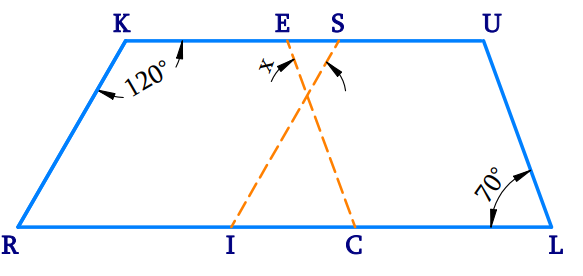# Ex.3.3 Q9 Understanding Quadrilaterals Solution-Ncert Maths Class 8

Go back to  'Ex.3.3'

## QuestionIn the above figure both $$RISK$$ and $$CLUE$$ are parallelograms. Find the value of $$x$$.

Video Solution
Ex 3.3 | Question 9

## Text Solution

What is Known?

In the given figure $$RISK$$and $$CLUE$$ are parallelograms.

What is Unknown?

Values of $$x$$

Reasoning:

The diagonals of a parallelogram bisect each other. Also, in a parallelogram, opposite angles are equal and adjacent angles are supplementary. Using this property, we can calculate the unknown angles.

Steps:In parallelogram $$RISK$$

$${\rm{RKS }} + \angle {\rm{ISK }} = {\rm{ 18}}0^\circ$$  (Adjacent angles of a parallelogram are supplementary)

\begin{align}{\rm{12}}0^\circ + \,\angle {\rm{ISK }} &= {\rm{18}}0^\circ \\\angle {\rm{ISK }} &= {\rm{ 6}}0^\circ\end{align}

\begin{align}{\angle {\text{I}}}&{ = \angle {\rm{K}}}\,{\left( \begin{array}{l}{\text{In parallelogram opposite }}\\{\text{angles are equal}}\end{array} \right)}\\&{ = {\rm{12}}{0^\circ }}&\end{align}

In parallelogram $$CLUE$$

\begin{align}{\angle {\rm{L}}}&{ = \angle {\rm{E}}}\,{\left( \begin{array}{l}{\text{In parallelogram opposite}}\\{\text{ angles are equal}}\end{array} \right)}\\&{ = {\rm{7}}{0^\circ }}&\end{align}

The sum of the measures of all the interior angles of a triangle is $$180^\circ.$$

\begin{align}x+{{60}^{\circ}}+{{70}^{\circ}}&={{180}^{\circ}} \\x+130&={{180}^{\circ}} \\x&={{180}^{\circ}}-{{130}^{\circ}} \\x&={{50}^{\circ}} \\\end{align}

Learn from the best math teachers and top your exams

• Live one on one classroom and doubt clearing
• Practice worksheets in and after class for conceptual clarity
• Personalized curriculum to keep up with school